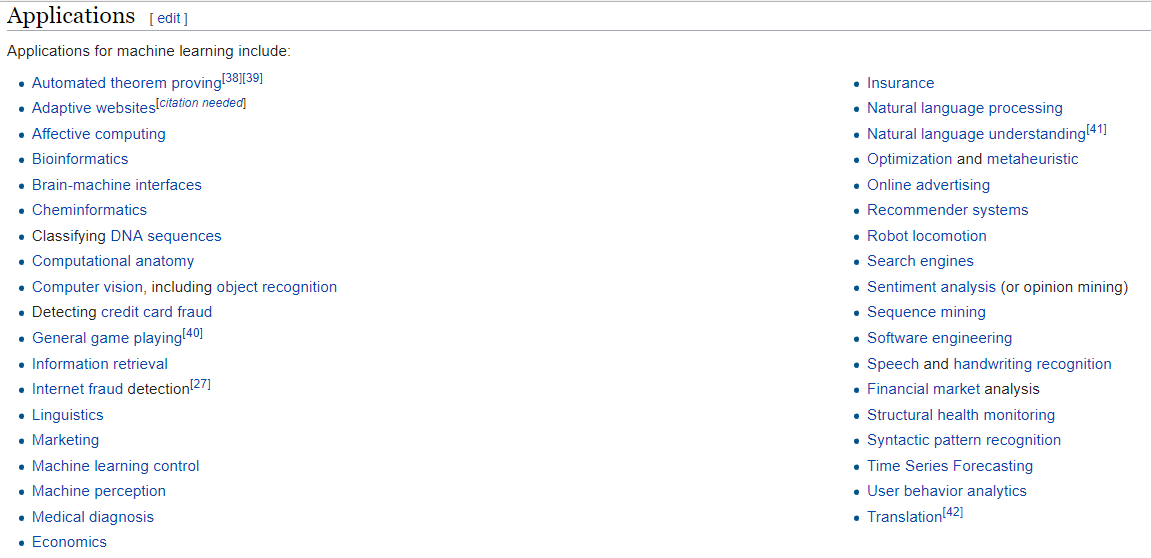[Quora] Which are the different usage fields of machine learning, deep learning and neural networks?

in ai •  2 years ago

First things first, let’s define machine learning, deep learning, and neural networks.

Machine learning algorithms are a class of algorithms that improve in performance with experience (more data).

Neural networks is a subclass of machine learning algorithms that are loosely inspired by the brain, and tries to learn a set of weights on neurons with experience to minimize a loss function.

Deep learning is a type of neural network where you have multiple layers of neurons; that is (in the simplest form) the output of a smaller neural network feeds into a new neural network and the learning is run again. The middle layers of neurons are often called “hidden layers” which is why you often hear deep learning neural networks containing X number of hidden layers.

There’s a host of different types of neural networks, and deep neural networks, but for the purpose of above explanation, I’ve assumed simple feed forward networks.

What are the different use cases?

As is evident from the definition, neural networks, and deep neural networks can both be applied to the same class of problems as any machine learning algorithm. This is because neural networks, and deep neural networks are subclasses of a larger class of algorithms.

But, you say, where are the different algorithms most effective?

Today, most tasks have seen great success from deep learning, and, correct me if I’m wrong, I believe that the current state of the art on most [read: all] better known AI problems use some form of deep learning somewhere.

Deep neural networks can, somewhat naïvely, be thought of as ordinary neural networks on steroids as they can generally do everything a “shallow” neural network can do just better and more advanced.

One thing to remember, however, is that the deeper the network, the more prone to overfitting it is (because it can encode more information), so you’ll need more data to train on which it.

Other machine learning algorithms have in recent years fallen in popularity due to the effectiveness, and elegance of neural networks.

Many are still good, and adequate for a range of problems, but the more advanced your problem, the more likely it is you want to you deep learning it seems. (at least until something better comes around).

What problems can machine learning solve today?

There are a lot of problems for which machine learning is currently used. Copying some of the fields in which it’s a applied from Wikipedia gets you:Source: